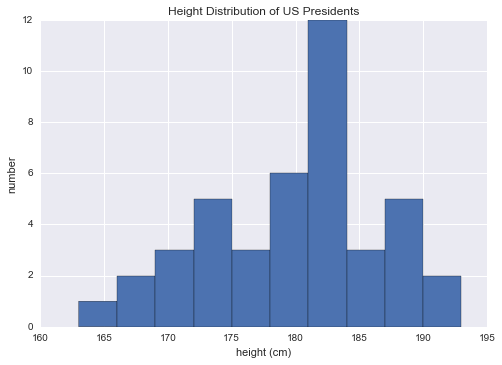## 9.6 聚合：最小、最大和之间的任何东西

NumPy 具有内置的快速的聚合函数，可用于处理数组；我们将在这里讨论和演示其中的一些内容。

### 对数组中的值求和

``````import numpy as np

L = np.random.random(100)
sum(L)

# 55.61209116604941
``````

NumPy 的`sum`函数的语法非常相似，结果在最简单的情况下是相同的：

``````np.sum(L)

# 55.612091166049424
``````

``````big_array = np.random.rand(1000000)
%timeit sum(big_array)
%timeit np.sum(big_array)

'''
10 loops, best of 3: 104 ms per loop
1000 loops, best of 3: 442 μs per loop
'''
``````

### 最小和最大

``````min(big_array), max(big_array)

# (1.1717128136634614e-06, 0.9999976784968716)
``````

NumPy 的相应函数具有相似的语法，并且同样运行得更快：

``````np.min(big_array), np.max(big_array)

# (1.1717128136634614e-06, 0.9999976784968716)

%timeit min(big_array)
%timeit np.min(big_array)

'''
10 loops, best of 3: 82.3 ms per loop
1000 loops, best of 3: 497 μs per loop
'''
``````

``````print(big_array.min(), big_array.max(), big_array.sum())

# 1.17171281366e-06 0.999997678497 499911.628197
``````

#### 多维聚合

``````M = np.random.random((3, 4))
print(M)

'''
[[ 0.8967576   0.03783739  0.75952519  0.06682827]
[ 0.8354065   0.99196818  0.19544769  0.43447084]
[ 0.66859307  0.15038721  0.37911423  0.6687194 ]]
'''
``````

``````M.sum()

# 6.0850555667307118
``````

``````M.min(axis=0)

# array([ 0.66859307,  0.03783739,  0.19544769,  0.06682827])
``````

``````M.max(axis=1)

# array([ 0.8967576 ,  0.99196818,  0.6687194 ])
``````

#### 其它聚合函数

NumPy 提供了许多其他聚合函数，但我们不会在这里详细讨论它们。

`np.sum` `np.nansum` 计算元素的和
`np.prod` `np.nanprod` 计算元素的积
`np.mean` `np.nanmean` 计算元素的均值
`np.std` `np.nanstd` 计算标准差
`np.var` `np.nanvar` 计算方差
`np.min` `np.nanmin` 寻找最小值
`np.max` `np.nanmax` 寻找最大值
`np.argmin` `np.nanargmin` 寻找最小值的下标
`np.argmax` `np.nanargmax` 寻找最大值的下标
`np.median` `np.nanmedian` 计算元素的中值
`np.percentile` `np.nanpercentile` 计算元素的百分位数
`np.any` N/A 计算是否任何元素是真
`np.all` N/A 计算是否所有元素是真

### 示例：美国总统的平均身高是多少？

NumPy 中可用的聚合对于汇总一组值非常有用。举个简单的例子，让我们考虑所有美国总统的身高。此数据位于`president_heights.csv`文件中，该文件是一个简单的逗号分隔的标签和值的列表：

``````!head -4 data/president_heights.csv

'''
order,name,height(cm)

1,George Washington,189

3,Thomas Jefferson,189
'''
``````

``````import pandas as pd
heights = np.array(data['height(cm)'])
print(heights)

'''
[189 170 189 163 183 171 185 168 173 183 173 173 175 178 183 193 178 173
174 183 183 168 170 178 182 180 183 178 182 188 175 179 183 193 182 183
177 185 188 188 182 185]
'''
``````

``````print("Mean height:       ", heights.mean())
print("Standard deviation:", heights.std())
print("Minimum height:    ", heights.min())
print("Maximum height:    ", heights.max())

'''
Mean height:        179.738095238
Standard deviation: 6.93184344275
Minimum height:     163
Maximum height:     193
'''
``````

``````print("25th percentile:   ", np.percentile(heights, 25))
print("Median:            ", np.median(heights))
print("75th percentile:   ", np.percentile(heights, 75))

'''
25th percentile:    174.25
Median:             182.0
75th percentile:    183.0
'''
``````

``````%matplotlib inline
import matplotlib.pyplot as plt
import seaborn; seaborn.set()  # 设置绘图风格

plt.hist(heights)
plt.title('Height Distribution of US Presidents')
plt.xlabel('height (cm)')
plt.ylabel('number');
``````